## A ball resting on a roof 75 meters high has 1000 Joules of gravitational potential energy. Calculate the mass of the ball. (SHOW ALL WORK)

Question

A ball resting on a roof 75 meters high has 1000 Joules of gravitational potential energy. Calculate the mass of the ball. (SHOW ALL WORK)

in progress 0
2 weeks 2021-09-02T20:20:21+00:00 1 Answers 0 views 0

The mass of the ball is 1.360 kilograms.

Explanation:

By Work-Energy Theorem, gravitational potential energy (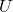), in joules, is the product of weight of the ball (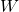), in newtons, and height (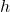), in meters. Please notice that weight is the product of the mass of the ball (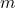) and gravitational acceleration (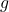), in meters per square second. Then, the formula for the mass of the ball is: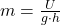(1)

If we know that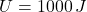,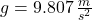and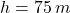, then the mass of the ball is: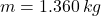The mass of the ball is 1.360 kilograms.